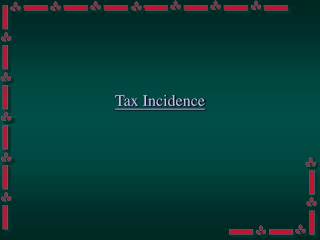DownloadDownload PresentationTax Incidence

# Tax Incidence

Télécharger la présentation## Tax Incidence

- - - - - - - - - - - - - - - - - - - - - - - - - - - E N D - - - - - - - - - - - - - - - - - - - - - - - - - - -
##### Presentation Transcript

1. Tax Incidence

2. t P Per unit tax • If a per unit tax t is imposed on a good, the consumer has to pay an extra amount of t for every unit of the good bought.

3. S1 P S0 t t t t Q Per unit tax • To the consumer, the supply curve is moved up by a vertical distance of t.

4. P S0 P1 P1 D Q Q1 Per unit tax • Before the tax is imposed, the consumer is paying P1 for every unit of the good.

5. S1 P S0 t P1 D Q Per unit tax • When t is imposed, the consumer has to pay more than before for each unit of the good. Q1

6. P S0 t P1 D Q Per unit tax • The consumer will therefore buy less of the good (the Law of Demand). S1 Q1

7. P S0 t P1 D Q Per unit tax • The consumer will therefore buy less of the good (the Law of Demand). S1 Q1

8. P S0 t P1 D Q Per unit tax • The consumer will therefore buy less of the good (the Law of Demand). S1 Q1

9. P S0 t P1 D Q Per unit tax • The consumer will therefore buy less of the good (the Law of Demand). S1 Q1

10. P S0 P1 D Q Per unit tax • The consumer will therefore buy less of the good (the Law of Demand). S1 t Q1 Q2

11. P S0 P2 P1 D Q Per unit tax • The consumer now pays P2 for each unit of the good. S1 t Q1 Q2

12. S1 P S0 t P2 P1 D Q Q1 Q2 Per unit tax • P2 may be less than the sum of P1 and t.

13. t P1 P2 Per unit tax • P2 may be less than the sum of P1 and t.

14. t P2 Per unit tax • P2 may be less than the sum of P1 and t. P1

15. t P1 P2 Per unit tax • P2 may be less than the sum of P1 and t.

16. P2 P1 t Per unit tax • P2 may be less than the sum of P1 and t.

17. P2 P1 t Per unit tax • P2 may be less than the sum of P1 and t.

18. t Tax incidence • In this case, the tax burden is said to be shared among the consumer and the producer.

19. t Tax incidence • In this case, the tax burden is said to be shared among the consumer and the producer.

20. t Tax incidence • In this case, the tax burden is said to be shared among the consumer and the producer.

21. t Tax incidence • In this case, the tax burden is said to be shared among the consumer and the producer.

22. t Tax incidence • In this case, the tax burden is said to be shared among the consumer and the producer.

23. t Tax incidence • In this case, the tax burden is said to be shared among the consumer and the producer.

24. Tax incidence • In this case, the tax burden is said to be shared among the consumer and the producer.

25. P S0 x P2 P1 D Q Tax incidence • For each unit of good, the consumer pays an extra amount of x. S1 t Q1 Q2

26. P S0 y P2 P1 D Q Tax incidence • For each unit of good, the producer receives P3, which is less than P1 by y. S1 t P3 Q1 Q2

27. Summary

28. S1 P S0 P2 P1 P3 D Q Q1 Q2 • After imposition of the per unit tax (t), for each unit of the good, the consumer pays more (P2) while the producer receives less (P3).

29. S1 P S0 P2 P1 t P3 D Q Q1 Q2 • The gap between the two amounts equals to the amount of the tax (t).

30. S1 P S0 P2 x P1 t y P3 D Q Q1 Q2 • The burden of the tax (t) is shared among the consumer (x) and the producer (y).

31. P Consumer’s Share x y Producer’s Share Q • The burden of the tax (t) is shared among the consumer (x) and the producer (y). Total Tax Burden S1 S0 P2 P1 t P3 D Q1 Q2

32. S1 P S0 Consumer’s Share P2 x P1 t y Producer’s Share P3 D Q Q1 Q2 • The distribution of the tax incidence between the consumer and the producer depends on the price elasticities of demand and supply.

33. Activities • With the help of supply and demand diagrams, show how the tax incidence is distributed when • demand is elastic and supply is inelastic • demand is inelastic and supply is elastic • demand is perfectly elastic • demand is perfectly inelastic • supply is perfectly elastic • supply is perfectly inelastic

34. Humanities Unit Curriculum Development Institute